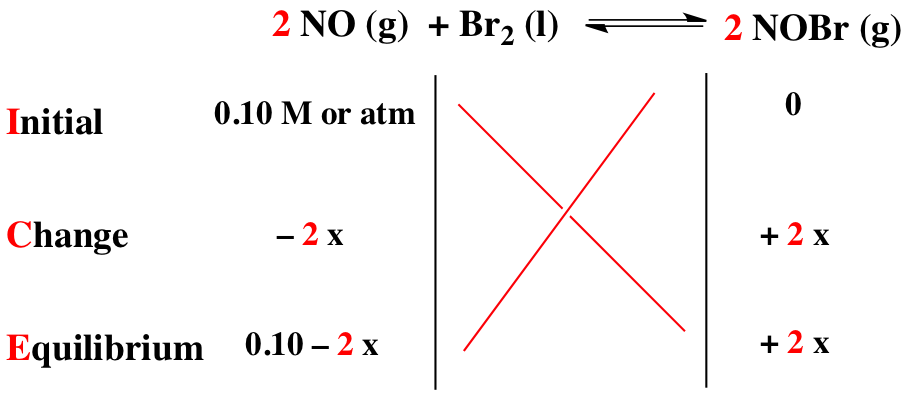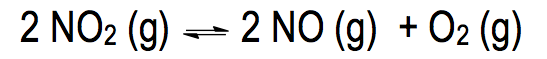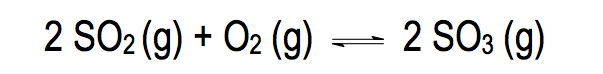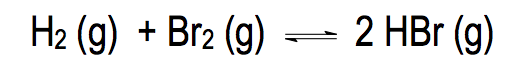Clutch Prep is now a part of Pearson
Ch.14 - Chemical EquilibriumWorksheetSee all chapters

# ICE Chart

See all sections
Sections
Chemical Equilibrium
ICE Chart
Le Chatelier's Principle
The Reaction Quotient
Equilibrium Expressions

In order to calculate the equilibrium concentrations of compounds we need to use an ICE Chart.

###### Calculating Equilibrium Concentrations

Concept #1: Understanding an ICE Chart

Transcript

Hey guys! In this new video, we're going to do something incredibly important – calculating equilibrium concentrations. Up to this point, I've been giving you questions in which our reaction is at equilibrium and the numbers I’m giving you are equilibrium amounts.
Now we’re going to be tasked with figuring out what those equilibrium amounts when I give you just initial concentrations. Remember, initial concentrations and equilibrium concentrations are totally different. We're going to say sometimes we’ll be asked to calculate concentrations at equilibrium after being given initial concentrations. We're going to say in order to do this, we're going to have to use our favorite friend, the ICE chart. Remember, what does ICE stand for? ICE stands for initial, change, equilibrium. We're going to learn how to use an ICE chart and when do we use an Ice chart. What we should realize here is that ICE charts are only allowed to have two types of units. We're going to say they're used to having atmospheres or molarity as the units. Remember, why those two units? Because atmospheres are connected to Kp and molarity is connected to Kc. We're still going to be dealing with our equilibrium constants because they go hand in hand with our ICE charts.
When do we use an ICE chart? You only ask yourself one question or really you say one thing. You're going to say anytime we have more than one compound in our balanced equation without an equilibrium concentration, then we have to use an ICE chart. Before we start this next question, what do I mean by this? In the next question we have three compounds within our balanced equation. Let's say I gave you the equilibrium amounts for two of them. We’d only be missing one person at equilibrium. In that case, we wouldn't need to do an ICE chart. But let's say I gave you only one person at equilibrium.
Technically we’d have to do an ICE chart in order to organize our work to see what the correct answer would be. Again, if you're missing more than one compound at equilibrium, you should use an ICE chart. If you don't have anyone at equilibrium, then you should definitely use an ICE chart.

An ICE Chart should be used when we are missing more than one equilibrium amount for compounds in our balanced equation.Since our equilibrium constant K is involved in an ICE Chart then we must continue to ignore solids and liquids.Example #1: We have a solution where Ag(CN)2 (g), CN (g), and Ag+ (g) have an equilibrium constant, K, equal to 1.8 x 10-19. If the equilibrium concentrations of Ag(CN)2 and CN are 0.030 and 0.10 respectively, what is the equilibrium concentration of Ag+?

Transcript

Let's take a look at the first two examples and see how we approach these types of questions. We say here when we have a solution where Ag(CN)2- gas, CN- gas, and Ag+ gas and they have an equilibrium constant K equal to 1.8 times 10 to the negative 19. If the equilibrium concentrations of Ag(CN)2- and CN- are these numbers respectively, what is the equilibrium concentration of Ag+?
We ask ourselves, do we use an ICE chart or not? Here I give you the equilibrium amounts of this compound, which is 0.030 and this compound which is 0.10. You're missing only one equilibrium amount. If you're missing only one equilibrium amount, then you don't have to do an ICE chart. We're simply going to say in this case that K equals products over reactants. We say that products here would be CN- squared times Ag+. Since we're going to need some room guys, I'm going to remove myself from the image so we have more room to work with. Divided by Ag(CN)2-.
What we're going to do now is we're going to plug in the numbers that we know. We know what K is. It's 1.8 times 10 to the negative 19. CN- we said was 0.10 and it's going to be squared. Ag+ is what we're looking for, so we're going to have it as a variable, divided by Ag(CN)2- which is 0.030. This question becomes fairly easy. All we have to do now is isolate our Ag+. What we're going to do first is multiply both sides by 0.030. These two multiply together to give us a new answer of 5.4 times 10 to the negative 21. That equals 0.10 squared times Ag+. We just want Ag+ so we're going to divide it out, the 0.10 squared. Our final answer at the end for silver ion will be 5.4 times 10 to the negative 19 molar.
Again, why didn't we have to use an ICE chart? We didn't have to use an ICE chart because we're missing only one person at equilibrium. When you're missing no one at equilibrium or you're missing only one variable at equilibrium, then you don't need to do an ICE chart. We only do an ICE chart when more than one concentration is missing at equilibrium.Example #2: We place 2.5 mol of CO and 2.5 mol of CO3 in a 10.0 L flask and let the system come to equilibrium. What will be the final concentration of CO2?  K = 0.47

Practice: For the reaction: N2 (g) + 2 O2 (g) ⇌ 2 NO2 (g), Kc = 8.3 x 10 -10 at 25°C. What is the concentration of N2 gas at equilibrium when the concentration of NO2 is twice the concentration of O2 gas?Example #3: When 0.600 atm of NO­­2 was allowed to come to equilibrium the total pressure was 0.875 atm. Calculate the Kof the reaction.

Transcript

Hey guys! In this brand-new video, we're going to continue with our conversation on calculating equilibrium concentrations. Let's take a look at this particular question. We're going to say when 0.600 atmospheres of NO2 was allowed to come to equilibrium the total pressure was 0.875 atmospheres. Calculate the Kp of the reaction.
All I'm giving you is 0.600 atmospheres of NO2. I'm saying I'm allowing it to come to equilibrium. What that tells me is this amount is not my equilibrium amount. It's rather my initial amount, so this is my initial pressure. All we say to ourselves is do we have to use an ICE chart? The answer here is yes, because we're going to have this missing an equilibrium amount, this is missing an equilibrium amount and this is missing an equilibrium amount. Anytime more than one of my compounds in my balanced equation is missing an equilibrium amount, I have to do an ICE chart. This number here, I'm giving it time to get to equilibrium which means it's my initial pressure. We're going to say I is initial, C is change, E is equilibrium.
We're going to say initially we're starting out with 0.600 atmospheres. Remember, atmosphere goes with Kp. I don't tell us anything about NO or O2 so they're initially at zero. Remember the important phrase we've been saying, we're losing reactants to make products. This is going to be minus 2x because of the two and it's minus because it's a reactant. Plus 2x, the two there, that's why it’s plus 2x. Plus X bring down everything, 0.600 minus 2x plus 2x plus X.
What we should realize here is they're telling me what the total pressure is. This goes back to Henry's law, or actually not Henry's law but more importantly, Dalton's law. Dalton's law says that the total pressure that we experience is equal to the pressure of all of the gases added up. My total pressure is equal to the pressure of NO2 plus the pressure of NO plus the pressure of O2. At equilibrium, that's my equilibrium total pressure.
What we're going to do here is say at equilibrium, each of these gases is equal to each of these equations. At equilibrium, our total pressure is 0.875 atmospheres. At equilibrium, NO2 is 0.600 minus 2x. At equilibrium, NO is 2x. At equilibrium, O2 is just X. We're going to say that this negative 2 and this positive 2 basically cancel each other out. Our equation becomes 0.875 atmospheres equals 0.600 plus X. We need to isolate X here so we're going to subtract, 0.600, 0.600. X here equals 0.275 atmospheres.
Remember, this is Dalton's law. The total pressure we experience is the pressure from all of the gases added up together. Since this is an equilibrium total pressure, we use the equilibrium equations for each of the compounds. We just isolated what X is, so all we need to do is take this X answer, plug it in here,
plug it in here, plug it in here and we know each one of them at equilibrium. If we know each of them at equilibrium, then it's those equilibrium amounts that we plug in to Kp. Remember, Kp is just products over reactants so it's NO squared times O2 divided by NO2 squared because the two and the two here.
When we plug in the X in for NO2, we get 0.050 atmospheres. When we plug it in for NO, at equilibrium NO equals 2x. Plug in the X that we just found. That's NO. Then O2 is just equal to X at equilibrium so it’s that same exact number. Take all those numbers and plug it in. When you do all that, you'll get your Kp equal to 33.28. To do this question, you had to have a knowledge of Dalton's law. Remember that deals with gases, and then apply that concept to this new idea of an ICE chart. It's kind of taking a little bit of old information combining with some new information in order to obtain our Kp value.Example #4: An important reaction in the formation of acid rain listed below.

2 SO2 (g)   +  O2 (g)  ⇌  2 SO3 (g)

Initially, 0.023 M SO2 and 0.015 M O2 are mixed and allowed to react in an evacuated flask at 340 oC. When an equilibrium is established the equilibrium amount of SO3 was found to be 0.00199 M. Calculate the equilibrium constant, Kc, for the reaction at 340 oC.Example #5: If Kc is 32.7 at 300oC for the reaction below:

H2 (g) +  Br2 (g) ⇌  2 HBr (g)

What is the concentration of H2 at equilibrium if a 20.0 L flask contains 5.0 mol HBr initially?

Transcript

Hey guys, in this brand new video, we're going to continue with our conversation on calculating equilibrium concentrations.
So, let's take a look at this particular question. We're going to say when .600 atmospheres of NO2 was allowed to come to equilibrium the total pressure was .875 atmospheres. Calculate the Kp of the reaction. All I'm giving you is .600 atmospheres of NO2. I'm saying I'm allowing it to come to equilibrium. What that tells me is this amount is not my equilibrium amount. It's rather my initial amount. So, this is my initial pressure. All we say to ourselves is do we have to use an ICE chart? The answer here is yes, because we're going to have this missing an equilibrium amount. This is missing an equilibrium amount and this is missing an equilibrium amount. Anytime more than one of my compounds in my balance equation is missing an equilibrium amount, I have to do an ICE chart. This number here, I'm giving it time to get to equilibrium which means it's my initial pressure.
We're going to say I is initial, C is change, E is equilibrium. We're going to say initially we're starting out with .600 atmospheres. Remember, atmosphere goes with Kp. I don't tell us anything about NO or O2 so they're initially at zero. Remember the important phrase we've been saying. We're losing reactants to make products. This is going to be minus 2x because of the 2. It's minus because it's a reactant. Plus 2x, the 2 there that's why it’s plus 2x. Plus x. Bring down everything. 0600 minus 2x plus 2x plus x.
Now, what we should realize here is they're telling me what the total pressure is. This goes back to Henry’s law, or actually not Henry's law but more importantly Dalton's law. Dalton's law says that the total pressure that we experience is equal to the pressure of all of the gases added up. My total pressure is equal to the pressure of NO2 plus the pressure of NO plus the pressure of O2. At equilibrium, that's my equilibrium total pressure. What we're going to do here is say at equilibrium, each of these gases is equal to each of these equations. At equilibrium, our total pressure is .875 atmospheres. At equilibrium, NO2 is .600 minus 2x. At equilibrium, NO is 2x. At equilibrium, O2 is just x. We're going to say that this negative 2 and this positive 2 basically cancel each other out. Our equation becomes .875 atmospheres equals .600 plus X. We need to isolate x here so we're going to subtract .600, .600. x here equals .275 atmospheres.
Remember, this is Dalton's law. The total pressure we experience is the pressure from all of the gases added up together. Since this is an equilibrium total pressure, we use the equilibrium equations for each of the compounds. We just isolated what x is, so all we need to do is take this x answer, plug it in here, plug it in here, plug it in here and we know each of them at equilibrium. If we know each of them at equilibrium, then it's those equilibrium amounts that we plug in to Kp. Remember Kp is just products over reactants, so NO squared times O2. Divide it by NO2 squared because the 2 and the 2 here. So, when we plug in the x in for NO2, we get .050 atmospheres. When we plug it in for NO, at equilibrium NO equals 2x. Plug in the x so we just found, that's NO. Then is just equal to x at equilibrium so it’s that same exact number. Take all of those numbers and plug it in. When you do all that, you'll get your Kp equal to 33.28.
To do this question, you had to have a knowledge of Dalton's law. Remember, that deals with gases and then apply that concept to this new idea of an ICE chart. It's kind of taking a little bit of old information, combining with some new information in order to obtain our Kp value.

Practice: At a given temperature the gas phase reaction: N2 (g) + O2 (g) ⇌ 2 NO (g) has an equilibrium constant of 4.00 x 10 -15. What will be the concentration of NO at equilibrium if 2.00 moles of nitrogen and 6.00 moles oxygen are allowed to come to equilibrium in a 2.0 L flask.

Example #6: Consider the following reaction:

COBr(g)  ⇌  CO (g) + Br(g)

A reaction mixture initially contains 0.15 M COBr2. Determine the equilibrium concentration of CO if Kc for the reaction at this temperature is 2.15 x 10-3.